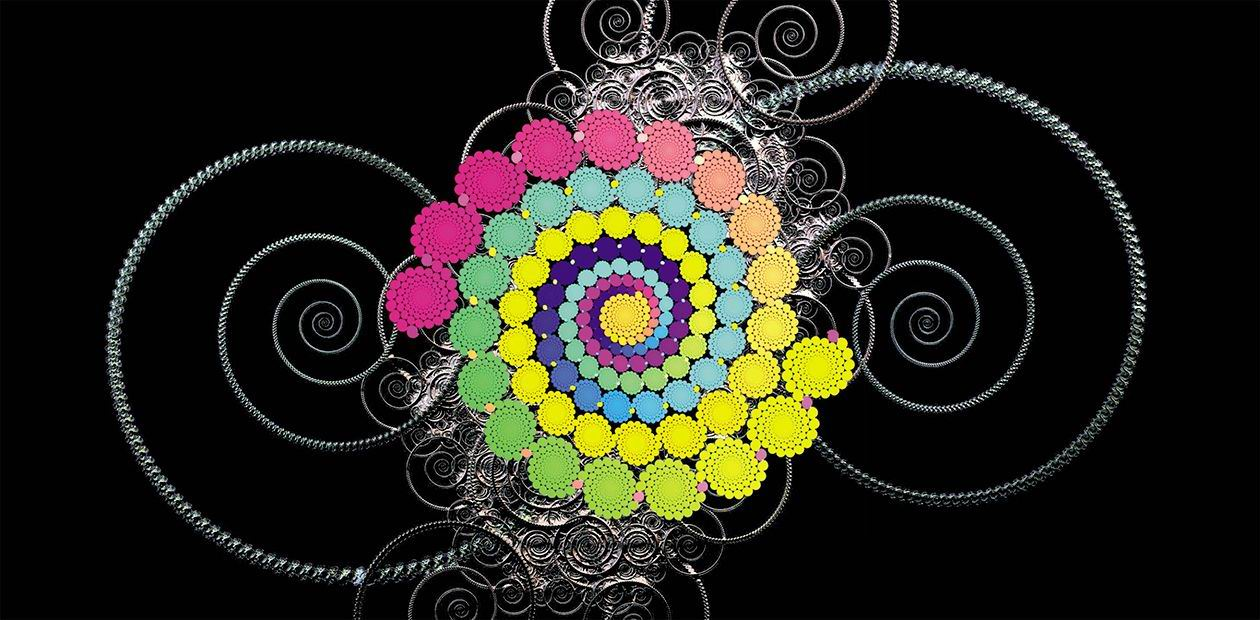• Authors
• Partners
• Students
• Libraries
• Contacts
• Language:900
Rubric: Live and Learn
Section: Mathematics# A Few Words about Fractals

This is not a page on needlework, as one could think at first glance. These pictures are unusual examples of self-similar sets, which form one of the simplest and easy-to-study classes of fractals...

Set K is called a self-similar set or an attractor of a system of contracting similarities {f1,…, fm} if K is a pool of its reduced copies K=f1(K)∪ ∪fm(K).

We can generalize this definition if we assume that we have several sets, e.g., K1,…, Kn, each of them composed of reduced copies of the same sets arranged in a certain order. In this case, the set of compact sets K1,…, Kn is called an attractor of a graph-oriented system of contracting similarities.

Geometry of self-similar sets is a branch of fractal geometry, which has made great progress in the last 25 years. These amazing mathematical objects will be described in more detail in the next issues of the journal.

All the images were constructed by the algorithm of beam tracking with the use of the novel code IFS Builder 3d developed by D. Mekhontsev and A. Kravchenko, Novosibirsk State University.

The publication was prepared by A. V. Tetenov, Candidate of Physics and Mathematics, Deputy Head

of the Mathematical Analysis Department, Gorno-Altaisk State University

Keywords